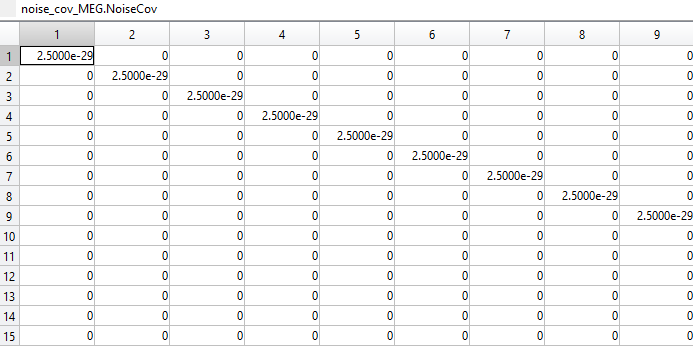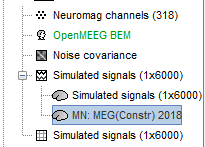# The covariance matrix and it is effect on the ImagingKernal

Dear All,

Hope you are doing well,

I want to compute the resolution matrix for meg and opm,
and as you know Resolution matrix= source.ImagingKernel * Gain;

I want to consider segma the noise variance as segma_opm = (40e-15)^2 for opm;
and segma_meg = (5e-15)^2 for meg;

So I can specify the noise level in brainstorm by specifying noise covariance matrix (S). If I just consider sensor noise (with uniform noise across sensors) then I can specify the noise covariance matrix to be S = sigma^2 I, where I is the Nsensors x Nsensors identity matrix and sigma^2 is the noise variance. For example, for OPMs sigma^2 would be (40 fT)^2.

So now I have two noise_covariance one for opm and another for opm:I imported different noise covariance and then I compute sources to check the Imaging Kernel if it is different then I saw them the same, so I have the same Imaging Kernel for different noise covariance matrix, so what should be the solution?